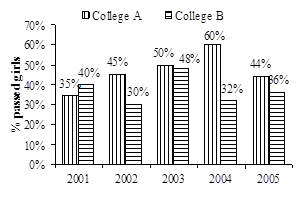# Quantitative Aptitude Questions 11th January 2019

January 11, 2019

### Quantitative Aptitude Questions 11th January 2019

Mathematics is one of the important sections of various Government exams, check out Daily Quant and data interpretation questions for preparation of various exams.

Q:A>Direction: (1-5):The following bar graph shows the percentage of boys and girls appeared for Class X exam for the period of five years 2005-2009. The line shows he total number of students appeared and the table shows the total students passed and percentage of boys and girls passed in the exam.1. What is the number of girls who failed in the year 2007?
(1) 75
(2) 80
(3) 85
(4) 90
(5) None of these

2. What is the sum of girls passed and boys failed in the year 2009?
(1) 546
(2) 556
(3) 566
(4) 576
(5) None of these

3. What is the difference between the total boys appeared and the total boys passed in the period of five years?
(1) 1146
(2) 1156
(3) 1166
(4) 1176
(5) None of these

4. What is the ratio of the total boys appeared in the year 2009 to the total girls passed in the year 2006?
(1) 7 : 5
(2) 7 : 4
(3) 7 : 3
(4) 7 : 2
(5) None of these

5. The number of boys passed in the year 2005 is what per cent more than the number of girls appeared in the year 2006?
(1) 10%
(2) 20%
(3) 25%
(4) 40%
(5) None of these

Directions (6-10): Following bar-graph shows the percentage of passed girls with respect to total passed students of two colleges A and B.1. If the number of boys passed from College A and College B is 220 and 175 respectively in the year 2002, then what is the difference between number of girls passed from A and B in year 2002?

(a) 75

(b) 80

(c) 95

(d) 105

(5) None of these

1. If the number of girls passed from College A and College B in year 2001 is equal to 70, then what is the sum of total number of passed students of College A and College B in the same year?

(a) 445

(b) 415

(c) 405

(d) 375

(5) None of these

1. If the number of girls passed from College A in year 2004 is equal to the number of boys passed from College B in year 2002 and it is 105, then what is the difference to total number of students passed from College A in 2004 and College B in year 2002?

(a) 25

(b) 30

(c) 35

(d) 40

(5) None of these

9.If total number of students passed from College A and College B in year 2005 is 400 and 500 respectively, then number of girls passed from College B is how much percent more than the number of girls passed from College A in the same year?

(a) 25/2%

(b)35/2%

(c)20/9

(d)8/7

(5) None of these

1. If the number of girls passed from College A and College B in year 2003 is 200 and 240 respectively, then number of boys passed from College B is what percent of number of boys passed from College A in same year?

(a) 80%

(b) 110%

(c) 120%

(d) 130%

(5) None of these

Q.A

1. 3
Total number of Girls who appeared in 2007 = 1000/100 * 36 = 360
Total number of Girls who passed in 2007 = 500/100 * 55 = 275
Total number of Girls who failed = 360 – 275 = 85
2. 1
Total number of girls passed in 2009 = 288
Total number of boys failed in 2009 = 770 – 512 = 258
Total sum = 288 + 258 = 546
3. 5
Total number of boys who appeared in all 5 years = 2925
Total number of boys who passed in all 5 years = 1739
Total difference = 2925 – 1739 = 1186
4. 3
Required Ratio = 770 : 330 = 7:3
5. 2
Number of boys passed in 2005 = 480
Number of girls appeared in 2006 = 400
Req % = (480 – 400)/400 * 100 = 20%
6. D
Total number of passed students of College A =(100*220)/55   = 400
Number of girls passed = 45% of 400 = 180
Total number of passed students of College= (100*175)70  = 250
Number of girls passed = 30% of 250 = 75
Difference = 180 – 72 = 105
7. D
For College A,
35% of girls = 70
Total student =(70*100)/35   = 200
For College B,
40% of girls = 70
Total student =  (70*100)/40 = 175
Sum = 200 + 175 = 375
8. A
Total students passed of College A in 2004 = 175
Total students passed of College B in 2002 = 150
Difference = 175 – 150 = 25
9. C
Number of girls passed of College= (44*400)/100  =176 Number of girls passed of College B =(36*500)/100 = 180
% difference =  [(180-176)/180]*100=20/9
10. D
Number of girls passed from College A = Number of boys passed from College A = 200
Number of girls passed from College B = 48% = 240
Total passed=(240/200)*48=500
Number of boys passed=52% of 500 = 260
%=(260/200)*100=130%